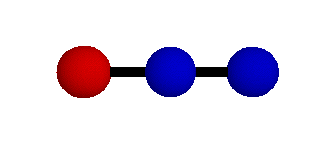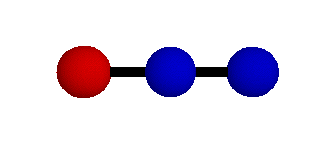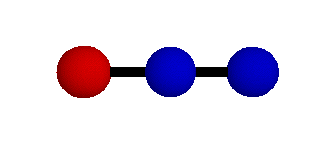## Characteristic Vibrations of N2O (Cv symmetry)

N2O (nitrous oxide) is a linear molecule with many similarities to CO2 (albeit with less symmetry). It is a minor contributor to the Earth's greenhouse effect. According to the IPCC, an increase of 46 ppb (17%) in the atmospheric abundance of N2O from 1750 to 2000 amounts to a radiative forcing ≈ 0.15 Watts/m2.
All three of the modes shown here are infrared-active to some degree, making them capable of absorbing and scattering infrared heat radiation from the Earth before it can escape to space.  The normal modes depicted below were modeled using hybrid density functional theory (B3LYP) and the cc-pVTZ basis set. Harmonic frequencies  calculated by Zúñiga et al. (2003, J. Mol. Spectrosc. 217:43-58) are also shown. These are based on a force-field model fitted to spectroscopic measurements.

### o2 = 596.3 cm-1 P Symmetry### o3 = 2282.2 cm-1 R+Symmetry